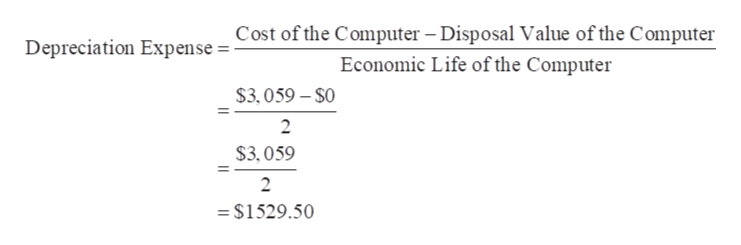# Firm X needs 1 computer.  Firm X can buy 1 computer for 3,059. The cost of debt for Firm X is 5%. The corporate tax equals 35%. Firm X follows a straight-line depreciation method and the economic life of the computer is 2 years. Compute the depreciation tax shield of year 2.

Question
9 views

Firm X needs 1 computer.  Firm X can buy 1 computer for 3,059. The cost of debt for Firm X is 5%. The corporate tax equals 35%. Firm X follows a straight-line depreciation method and the economic life of the computer is 2 years. Compute the depreciation tax shield of year 2.

check_circle

Step 1

Cost of the Computer = \$3,059

Economic Life of the Computer = 2 Years

Disposal Value of the Computer = \$0

Calculation of the depreciation expense is as follows:help_outlineImage TranscriptioncloseCost of the Computer Disposal Value of the Computer Depreciation Expense Economic Life of the Computer \$3,059-\$0 2 \$3,059 2 = \$1529.50 fullscreen
Step 2

As the Firm X uses the straight-line method for depreciation, then depreciation for both year is \$1529.50

Depreciation Expense of ...

### Want to see the full answer?

See Solution

#### Want to see this answer and more?

Solutions are written by subject experts who are available 24/7. Questions are typically answered within 1 hour.*

See Solution
*Response times may vary by subject and question.
Tagged in